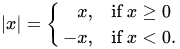# Increment/decrement a variable by a second variable so it gets closer to a third

Hi,
I need to increase/decrease 1st var by second var, so it will be closer to third var. I know, that this is possible by if, but is this possible on 1 line? (vars can be also -)

Uh, what are you trying to make? Elaborate please.

I have local a 1, b 2 and c 11, can i do that i will cont var, that is c ± b, closer to a

This is pretty simple, but I don’t see why this would need to be on one line.

For this example I will use X, Y, and Z as variables.

``````if x > z then
x = x - y
elseif x < z then
x = x + y
end
``````

Why do you want this to be done in 1 line? Doesn’t seem necessary when you can simply make a function.

1 Like

I need to do it many times, so the method with if is last solution. I thought if there is some math function.

Just create a function, using one line is not an alternative.

My internet died temporarily, late response.

It can be done on one line?
`x = ((x >= z) and (x - y)) or (x + y)`

`x` will be set to `x - y` if `x >= z` is true; if not it will be set to `x - y`

2 Likes

Consider reading this short stackoverflow page and mozilla webpage on how exactly that works.

They’re both useful resources.

so any other number than 0 is logical true?

Oh that reminded me of a potential bug, thanks for mentioning that and I will update my original post to `x = ((x >= z) and (x - y)) or (x + y)`

why,and will execute first in both cases

Nevermind, you are right. If `x > z` is false then it will be caught by `x + y` for when `x == z`.lol, i made mistake, there must be or ((x<z)and(x+y))

Continuing with `a` as your subject, `b` as the delta and `c` as your point of interest such that either `a+b` or `a-b` is closer to `c`, and `a` will be turned into one of those terms, you can do

``````a = math.min(math.abs(c-(a+b)), math.abs(c-(a-b)))*math.abs(a)/a*math.abs(b)/b+c
``````

to avoid using conditional statements. It’s up to you to judge whether you like it more.
Note that my solution doesn’t work if either a or b are 0.

My description of the problem is based on my understanding of your initial question. Your reply and your accepted solutions seem to solutions to two more different problems, as far as I can tell.

1 Like

what does math.abs???

Absolute value of a number. `math.abs` based on the real-valued absolute value of any scalar:Basically, removes the sign of a number (any `math.abs(n)` is always positive). In this case, writing

``````math.abs(a)/a
``````

returns the sign of the number only (`|-9|/-9=-1, |9|/9=1`), and

``````math.abs(a-b)
``````

returns the absolute difference of two scalars. Imagine it as the “distance” between two points on the number line.

2 Likes

local result = x>=y and x-y or x<y and x-y